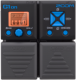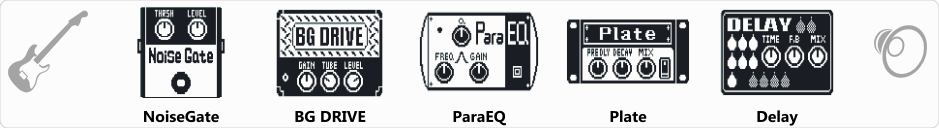# Bogner Solo

Discussion in 'Zoom G1on/G1Xon' started by Elvis Tavora, Mar 31, 2019.

1. Bogner SoloDevice: Zoom G1on
Firmware: 1.21

Name on device: Bogner Sol
Optimized for: Guitar Amp

Effects chain:..

Effect: "NoiseGate" (Dynamics / Filter), active - "yes"
"THRSH" = 10
"Level" = 100

Effect: "BG DRIVE" (Amp simulators), active - "yes"
"Gain" = 20
"Tube" = 32
"Level" = 97
"Trebl" = 10
"Middl" = 66
"Bass" = 47
"Prese" = 35
"CAB" = BGN DRIVE 4x12
"OUT" = LINE

Effect: "ParaEQ" (Dynamics / Filter), active - "yes"
"Freq1" = 125Hz
"Q1" = 1
"Gain1" = -5
"Freq2" = 8.0kHz
"Q2" = 1
"Gain2" = -4
"Level" = 100

Effect: "Plate" (Delay / Reverb), active - "yes"
"PreD" = 73
"Decay" = 51
"Mix" = 19
"Color" = 47
"LoDMP" = 85
"HiDMP" = 74
"Tail" = On
"Level" = 100

Effect: "Delay" (Delay / Reverb), active - "yes"
"Time" = 560
"F.B" = 30
"Mix" = 30
"HiDMP" = 10
"P-P" = P-P
"Level" = 100
"Tail" = Off

Patch Volume: 90

Note: This is a patch file, you will need to download and install the ToneLib-Zoom software to use the patch.

File size:
423 bytes
Views:
215